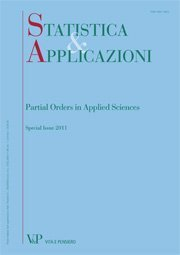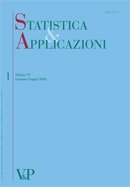Libri di Walid Ahmad Abu-Dayyeh - libri Statistica & Applicazioni

Department of Statistics - Yarmouk University Irbid, JORDAN
Share:

### Author's books

Maximum likelihood estimator of the shape parameter of the weibull distribution using ranked set sampling digitalformat:Article| STATISTICA & APPLICAZIONI - 2013 - 2
Year: 2014
In this paper, we will consider the (maximum likelihood estimators) MLEs of the shape parameter p of the Weibull distribution...
Estimation of the variance for logistic distribution under ranked set sampling and simple random sampling: a comparative study
Free
digitalformat:Article| STATISTICA & APPLICAZIONI - 2012 - 2
Year: 2012
SUMMARY The logistic distribution is applicable in many area of research. In this study, several estimators of the variance when the location parameter is known and unknown are considered when data are gathered under simple random sampling (SRS) and ranked set sampling (RSS). For some estimators considered, the bias and mean square error (MSE) are not gotten in closed form. Using Monte Carlo simulations, comparison of these estimators is made based on biases, MSE and efficiency. When the estimators are compared, it is found that estimators based on maximum likelihood method are more efficient than other estimators considered, under both SRS and RSS. However, estimators based on RSS have more advantages over those based on SRS. Keywords: Logistic Distribution, Ranked set Sampling, Simple Random Sampling, Variance, Estimations.
Estimation of  = P(X>Y) using ranked set sampling
Free
digitalformat:Article| STATISTICA & APPLICAZIONI - 2010 - 2
Year: 2010
SUMMARY Nonparametric-type estimation of  ¼ PðX > YÞ, based on ranked set sampling (RSS) technique with concomitant random variable, is considered. The maximum likelihood estimation (MLE) of  is also considered using RSS and median ranked set sampling (MRSS) techniques with concomitant random variable. The estimators obtained are compared to their counterparts based on simple random sampling (SRS) using bias and mean square error (MSE). It appears that the suggested nonparametric estimator based on RSS is more efficient than that based on SRS and the MLE based on MRSS is more efficient than the MLE based on RSS which is in turn more efficient than the MLE based on SRS. Keywords: Simple Random Sampling, Ranked Set Sampling, Median Ranked Set Sampling, Nonparametric Estimation; Maximum Likelihood Estimation, Mean Square Error. RIASSUNTO La stima non parametrica di  ¼ PðX > YÞ basata sulla tecnica del Ranked Set Sampling (RSS) con variabile concomitante e` considerata preliminarmente. Successivamente viene trattata la Stima di Massima Verosimiglianza (MLE) di  nel caso Normale usando sia RSS sia il Median Ranked Set Sampling (MRSS) con variabile concomitante. Gli stimatori ottenuti sono confrontati con quelli basati sul Simple Random Sample (SRS) usando il bias e l’errore quadratico medio (MSE). Emerge che lo stimatore non parametrico proposto basato su RSS e`piu` efficiente di quello basato su SRS e lo stimatore MLE basato su MRSS e`piu` efficiente di quello MLE basato su RSS che a sua volta risulta piu` efficiente di quello MLE basato su SRS.
Comparison of several confidence intervals for normal distribution with known coefficient of variation
Free
digitalformat:Article| STATISTICA & APPLICAZIONI - 2010 - 1
Year: 2010
In this paper, we consider the problem of constructing confidence intervals for the normal population mean when the coefficient of variation is known. We obtain 13 confidence intervals using different pivots. Also we conduct a simulation study to compare the expectations as well as the standard deviations of the intervals lengths. Keywords: Coefficient of Variation, Normal Distribution, Pivotal Method, Confidence Interval.

### Browse the archive

#### 2020 issues

This site or third-party tools used by this make use of cookies necessary for the operation and useful for the purposes outlined in the cookie policy.